Select Page

# CBSE MCQ in English Answers for Maths 12 Science Probability

CBSE MCQ in English Answers for Maths 12 Science Probability to enable students to get Answers in a narrative video format for the specific question.

Expert Teacher provides CBSE MCQ Answers for Maths 12 Science Probability through Video Answers in English language. This video solution will be useful for students to understand how to write an answer in exam in order to score more marks. This teacher uses a narrative style for a question from Probability not only to explain the proper method of answering question, but deriving right answer too.

Please find the question below and view the Answer in a narrative video format.

Question:

## Similar Questions from CBSE, 12th Science, Maths, Probability

Question 1 : One card is drawn from a pack of 52 cards. Find the probability of getting a red card:

Question 2 : In a group of 30 scientists workings on an experiment, 20 never commit error in their work and are reporting results elaborately. Two scientists are selected at random from the group. Find the probability distribution of the number of selected scientists who never commit error in work and reporting. Also find the mean of the distribution. What values are described in this question?  (View Answer Video)

Question 3 : A and B throw a pair of dice alternately. A wins the game if he gets a total of 9 and B wins if he gets a total of 7. If A starts the game, find the probability of winning the game by B.   (View Answer Video)

Question 4 :  Probability of solving specific problem independently by A and B are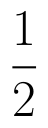and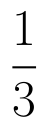respectively. If both try to solve the problem, independently, then find the probability that exactly one of them solves the problem.  (View Answer Video)

Question 5 : If P(A)=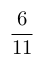, P(B)=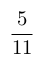and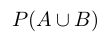=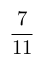, find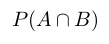. (View Answer Video)

### Continuity and Differentiability

Question 1 : Differentiate the function w.r.t.x. (View Answer Video)

Question 2 : Findfor the function. (View Answer Video)

Question 3 : If x and y are connected parametrically by the equation, without eliminating the parameter, find. (View Answer Video)

Question 4 : If, findin terms of y alone. (View Answer Video)

Question 5 :  Find the second order derivative of the function. (View Answer Video)

### Integrals

Question 1 : Evaluate :(View Answer Video)

Question 2 : Evaluate:as limit of sums.  (View Answer Video)

Question 3 : Find :(View Answer Video)

Question 4 : Evaluate :(View Answer Video)

Question 5 : Find the integral of the function. (View Answer Video)

### Matrices

Question 1 : Find the inverse of the matrix,. (View Answer Video)

Question 2 : Find the value of x from the equation:. (View Answer Video)

Question 3 : Compute:. (View Answer Video)

Question 4 : Given,, find the value of w. (View Answer Video)

Question 5 : Find the value of z,  from the equation:. (View Answer Video)